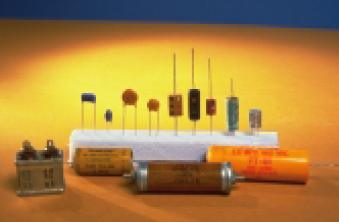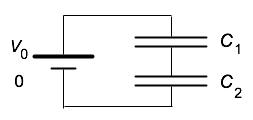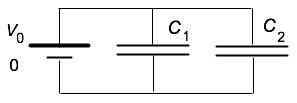• If you are citizen of an European Union member nation, you may not use this service unless you are at least 16 years old.

View

# Capacitors in series and parallel

last edited by 1 year, 3 months ago

8.4.P5

 A capacitor is a device for storing electrical energy in the form of a separation of opposite charges. Since these opposite charges attract and would naturally come together, there is energy available when they are separated. The energy can be obtained for conversion to other forms by allowing the separated charges to come together, their mutual attraction creating kinetic energy as they move together. A variety of these devices are shown at the right. (From Understanding Physics, Cummings, Laws, Redish, and Cooney: John Wiley & Sons, Inc., 2003) The magnitude of charge on each plate of the capacitor is related to the voltage difference across the plate and the capacitance, C, of the capacitor: Q = CΔV.If we connect the negative plate of one capacitor of capacitance Cto the positive plate of a second capacitor of capacitance C2, we say we are connecting them in series. If we connect the positive plate of one capacitor of capacitance C1 to the positive plate of a second capacitor of capacitance C2, we say we are connecting them in parallel.

 A. In the figure at the right are shown two capacitors connected in series. Assume that the capacitors were first connected to each other and then to the battery.   If the charge on the positive plate of capacitor C1 is Q1 (a positive charge), what is the charge on the negative plate of capacitor C1? On the positive plate of capacitor C2? On the negative plate of capacitor C2? Explain how you know. If the voltage rise in the battery is ΔV, what is the voltage drop across each of the individual capacitors? Explain how you know. Using your result above, find the effective capacitance of the combined pair of capacitors. (That is, what you would measure their capacitance as if they were contained in a box and you didn't know they were two separate capacitors.)In the figure at the right are shown two capacitors connected in parallel. Assume that the capacitors were first connected to each other and then to the battery. If the charge on the positive plate of capacitor C1is Q1 (a positive charge) and the charge on the positive plate of capacitor C2 is Q2 (a positive charge), what is the charge on the negative plate of capacitor C1? On the negative plate of capacitor C2? Explain how you know. If the voltage rise in the battery is ΔV, what is the voltage drop across each of the individual capacitors? Explain how you know. Find  Q1  and Q2 in terms of C1, C2, and V0.  Using your results above, find the effective capacitance of the combined pair of capacitors. (That is, what you would measure their capacitance as if they were contained in a box and you didn't know they were two separate capacitors.C. Can you relate the relationships you found above to how the capacitance depends on the shape (area and plate separation) of the capacitor? If you can, explain how. If you can't, explain why not.

Joe Redish 3/15/08